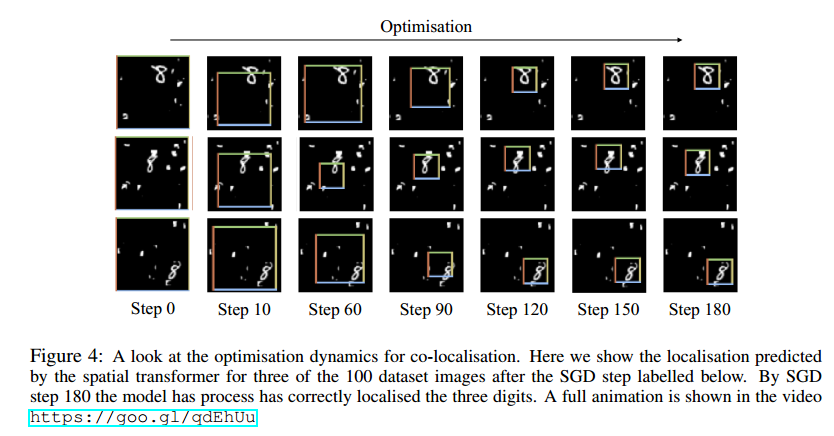# 1 简介

## 1.2 问题提出

CNN在图像分类中取得了显著的成效，主要是得益于 CNN 的深层结构具有 空间不变性（spatially invariance）（平移不变性，旋转不变性），所以图像上的目标物体就算是做了平移或者旋转，CNN仍然能够准确的识别出来，这对于CNN的泛化能力是有益的。

• 空间不变性主要是由于 Pooling 层 和 步长不为1的卷积层 的存在带来的。实际上主要是池化层的作用，因为大部分的卷积层的步长都是大于1而又小于卷积核大小的，也就是滑动时是有重叠的，而池化层一般不是重叠的。也就是说这些层越多，越深，池化核或卷积核越大，空间不变性也越强；但是随之而来的问题是局部信息丢失，所以这些层越多准确率肯定是下降的，所以主流的CNN分类网络一般都很深，但是池化核都比较小，比如2×2。
• 比如ResNet，GoogLeNet，VGG，FCN，这些网络的总降采样比例一般是 16或32，基本没有见过 64倍，128倍或者更高倍数的降采样（会损失局部信息降低准确率），也很少见到 2倍或者4倍的降采样比例（空间不变性太弱，泛化能力不好）。不过这个是跟数据集中的图像大小有关的，上述主流图像分类网络基本都是针对于 ImageNet数据集做分类的，ImageNet中的图片都比较大，一般在 256×256 左右。如果数据集中的图像本来就很小，那么降采样比例就也会小，比如 MNIST数据集，图像只有28×28，所以LeNet中的降采样比例是4。总之，降采样比例要根据数据集调整，找到合适的降采样比例，才能保证准确率的情况下，有较强的空间不变性。

## 1.2 解决方法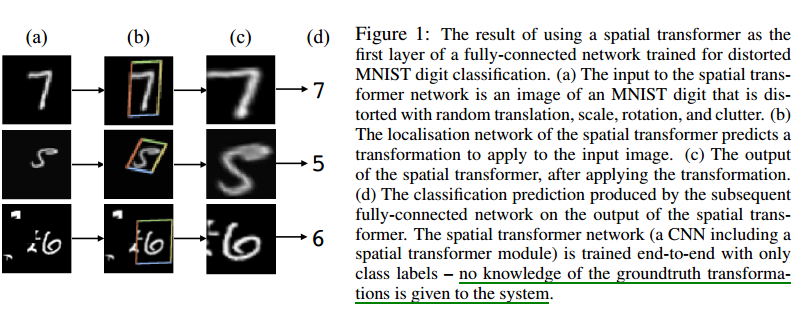• (a) ：输入图片
• (b) ：框起来的是用于后面网络进行进一步识别分类的部分，这一部分是就是Spatial Transformer的结果
• (c) ：输出层的可视化
• (d) ：预测结果

CNN是尽力让网络适应物体的形变，而STN是直接通过 Spatial Transformer 将形变的物体给变回到正常的姿态（比如把字摆正），然后再给网络识别。

• image classification ：如果数据集中的图像上的目标形变很大，噪声很大，位于图片中心较远，那么 Spatial Transformer 可以将物体部分 “剪裁” 出来，并做一定的旋转，缩放变换，使之成为大小统一的图片，便于后续网络识别，并且获得比CNN更好的结果。
• co-localisation ：给定输入图片，不确定是否有物体，如果有，可以使用Spatial Transformer做出定位。
• spatial attention ：对于使用attention机制的视觉任务，可以很轻松的使用 Spatial Transformer 完成。

# 2 Spatial Transformer结构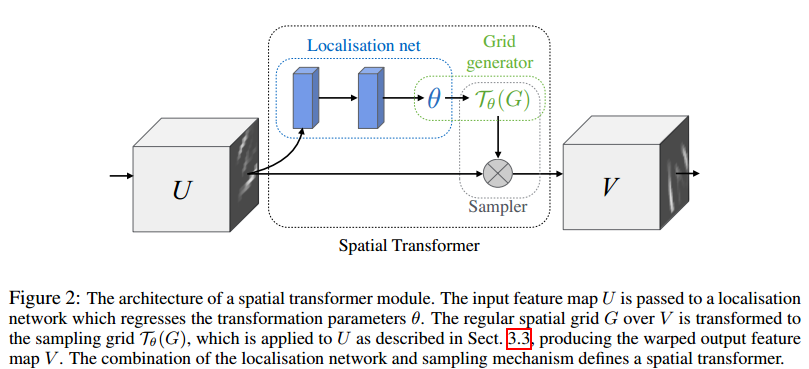• $U \in R^{H \times W \times C}$ 为输入 feature map
• $V \in R^{H’ \times W’ \times C’}$ 为输出 feature map
• $\theta=f_{loc}(U)$ 是一个回归子网络
• $T_{\theta}$ 表示以参数 $\theta$ 为变换矩阵的某种变换，可以是2维仿射变换(2D affine transformation )，平面投影变换(plane projective transformation )，薄板样条变换(thin plate spline )
• $G_i = (x^t_i, y_i^t)$ 代表V中的像素点 $G = \{G_i\}$ 是V中像素点的整体。
• $T_{\theta}(G)$ 代表下面图3中，输入U上的绿色区域的坐标。

## 2.2 Grid generator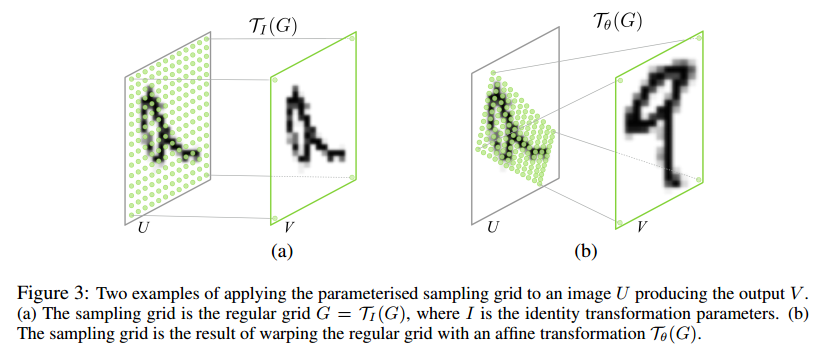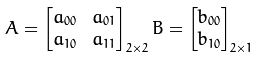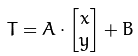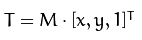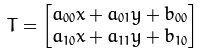• 已知 M 和 X，求T; 这个很简单，直接矩阵相乘。
• 已知 X 和 T , 求M; 可以选取三对点，带入上面的式子中，列方程，6个方程6个未知数；• $(x^t_i, y_i^t)$ are the target coordinates of the regular grid in the output feature map ，代表的是图3输出V中的像素点，即目标像素坐标；
• $(x^s_i, y_i^s)$ are the source coordinates in the input feature map that define the sample points ,代表的是图3输入U中被绿色点覆盖的像素点，即源像素坐标；
• $A_{\theta}$ is the affine transformation matrix ，代表的是仿射变换矩阵。其中的成员 $\theta_{ij}$ 由 localisation network 回归生成。图3或图2中的 $T_{\theta}$ 这时指的仿射变换 $A_{\theta}$。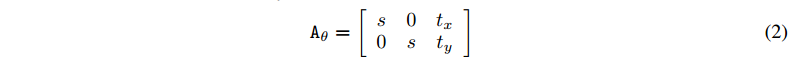## 2.3 Sampler

$(x^s_i, y_i^s)$ 是U中绿色区域的坐标，来看看更加具有一般性的采样问题如何描述：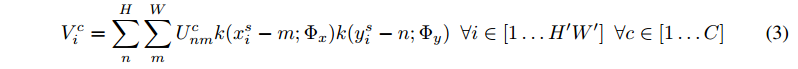• $U_{nm}^c$ 是输入feature map上第 $c$ 个通道上坐标为 $(n, m)$ 的像素值；
• $V_i^c$ 是输出 feature map上第 $c$ 个通道上坐标为 $(x^t_i, y_i^t)$ 的像素值；
• $k()$ 表示插值核函数；
• $Φx , Φy$ 代表 x 和 y 方向的插值核函数的参数；
• $H,W$ 输入U的尺寸;
• $H’,W’$ 输出 V 的尺寸;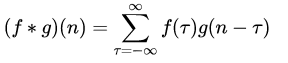• $f(\tau)$ 相当于 $U_{nm}^c$ ；
• $g(n-\tau)$ 相当于 $k(x_i^s-m; \Phi_x)$ 或 $(y_i^s-m; \Phi_y)$ 因为这里的卷积是 一维的。• 其中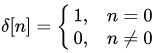• $\lfloor x + 0.5\rfloor$ 向下取整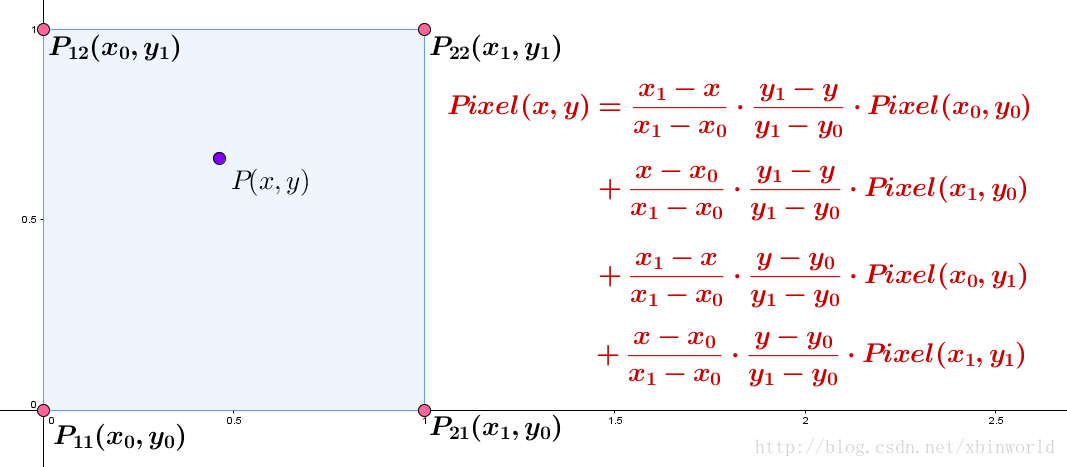## 2.4 前向传播

• 实际上首先进行的是 localisation network 的回归，产生 变换矩阵的参数 $\theta$ ，进而resize为 变换矩阵 $T_{\theta}$ ;
• 根据 V中的 目标坐标 $(x^t_i, y_i^t)$ 做逆向仿射变换变换到源坐标 $(x^s_i, y_i^s)$ ： $Source= T_{\theta} \cdot Target$ ， 源坐标 $(x^s_i, y_i^s)$ 位于U上；对应图中 1,2步；
• 在U中找到 源坐标 $(x^s_i, y_i^s)$ （小数坐标）附近的四个整数坐标，做双线性插值，插值后的值作为 目标坐标 $(x^t_i, y_i^t)$ 处的像素值；对应图中 3,4步；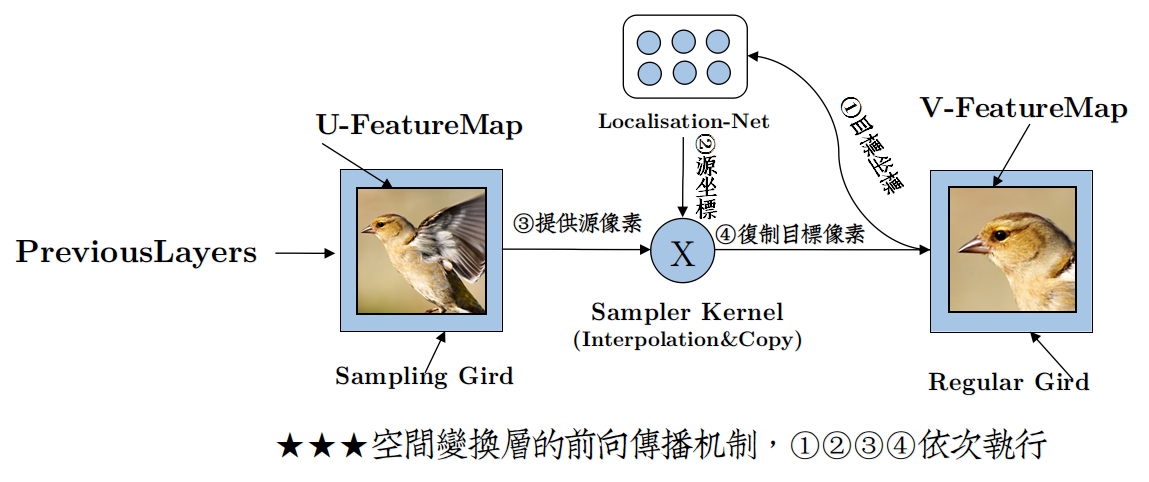## 2.5 梯度流动与反向传播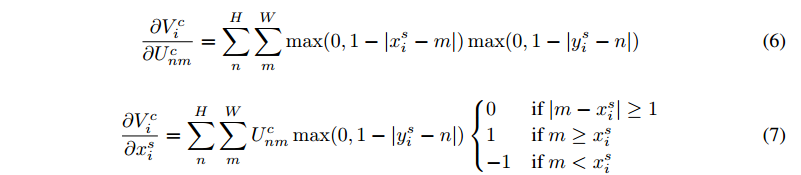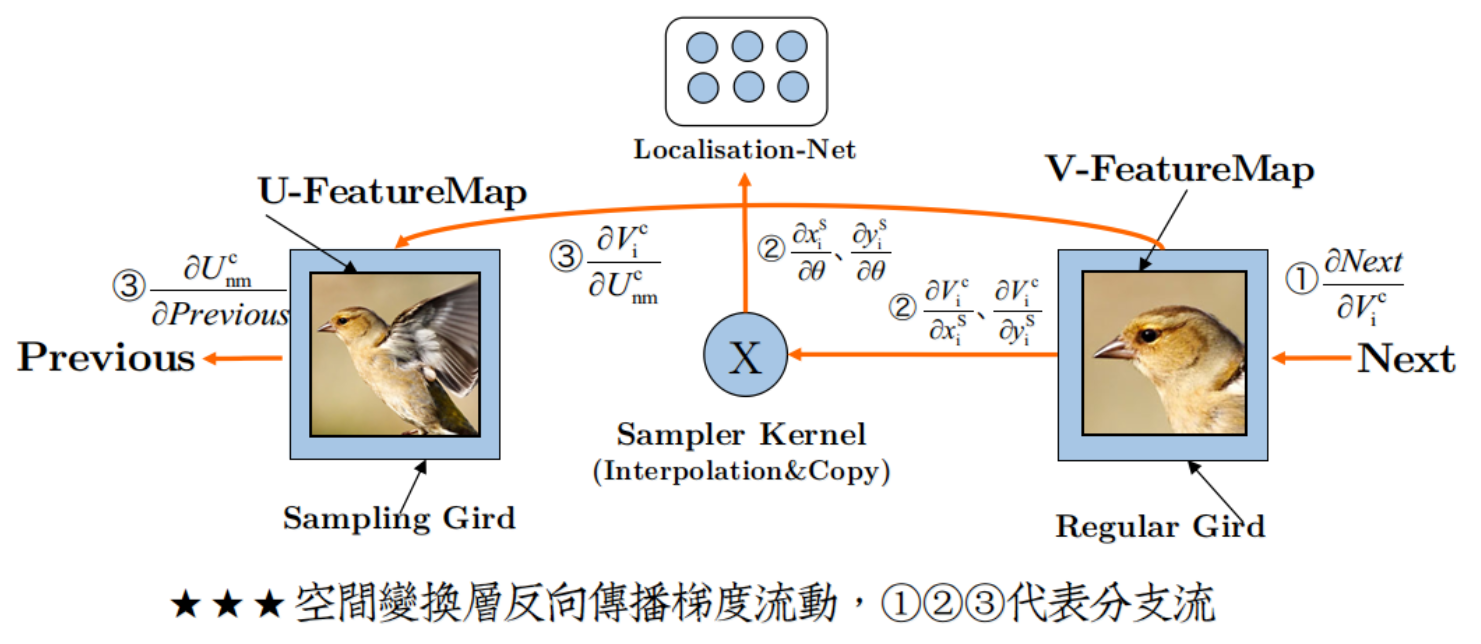# 3 试验

## 3.1 Distorted MNIST

• 旋转：rotation (R),
• 旋转+缩放+平移：rotation, scale and translation (RTS),
• 投影变换：projective transformation (P),
• 弹性变形：elastic warping (E) – note that elastic warping is destructive and can not be inverted in some cases.

• 仿射变换：affine transformation (Aff),
• 投影变换：projective transformation (Proj),
• 薄板样条变换：16-point thin plate spline transformation (TPS)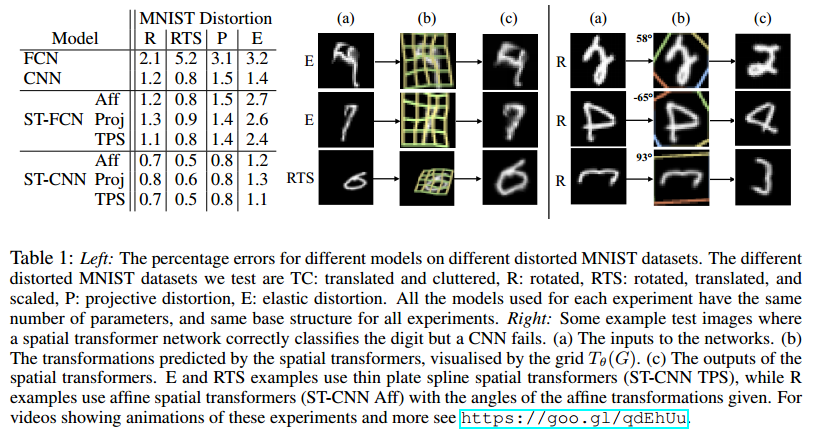• 左侧：不同的形变策略以及不同的 Spatial Transformer网络变种与 baseline的对比；
• 右侧：一些CNN分错，但是ST-CNN分对的样本
• (a)：输入
• (b)：Spatial Transformer层 的 源坐标（$T_{\theta}(G)$ ）可视化结果
• (c)：Spatial Transformer层输出
• 很明显：ST-CNN优于CNN, ST-FCN优于FCN，说明Spatial Transformer确实增加了 空间不变性
• FCN中由于没有 池化层，所以FCN的空间不变性不如CNN，所以FCN效果不如CNN
• ST-FCN效果可以达到CNN程度，说明Spatial Transformer确实增加了 空间不变性
• ST-CNN效果优于ST-FCN，说明 池化层 确实对 增加 空间不变性很重要
• 在 Spatial Transformer 中使用 plate spline transformation (TPS) 变换效果是最好的
• Spatial Transformer 可以将歪的数字扭正
• Spatial Transformer 在输入图片上确定的attention区域很明显利于后续分类层分类，可以更加有效地减少分类损失

FCN ，13.2% error； CNN ， 3.5% error； ST-FCN ，2.0% error； ST-CNN ，1.7% error.

## 3.2 Street View House Numbers

Street View House Numbers是一个真实的 街景门牌号 数据集，共200k张图片，每张图片包含1-5个数字 ，数字都有形变。

• baseline character sequence CNN model ：11层，5个softmax层输出对应位置的预测序列
• STCNN Single ：在输入层添加一个Spatial Transformer
• ST-CNN Multi ：前四层，每一层都添加一个Spatial Transformer 见下面 tabel 2 右侧
• localisation networks 子网络：两层32维的全连接层
• 使用仿射变换和双线性插值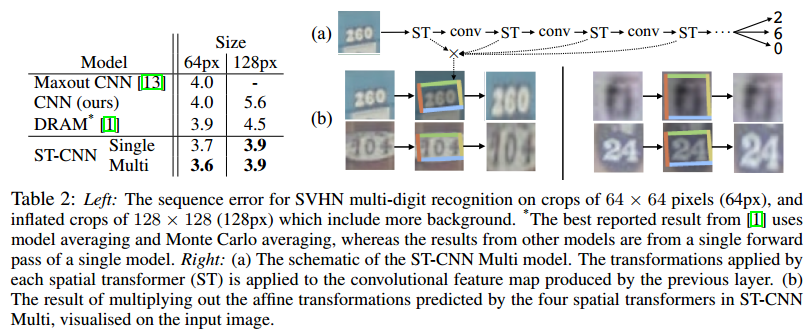## 3.3 Fine-Grained Classification

• baseline CNN model ： an Inception architecture with batch normalisation pre-trained on ImageNet and fine-tuned on CUB – which by itself achieves the state-of-the-art accuracy of 82.3% (previous best result is 81.0% ).
• ST-CNN, which contains 2 or 4 parallel spatial transformers, parameterised for attention and acting on the input image.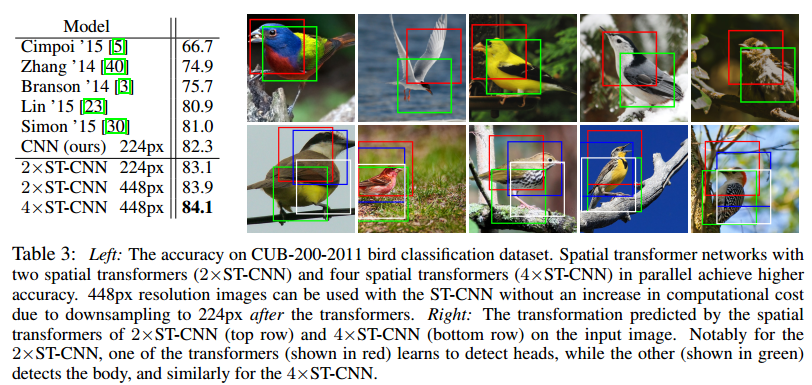• ST-CNN效果最好
• 右侧上边使用了 2 路 Spatial Transformer并行，可以看到其中一个 spatial transformer(红色) 检测的是鸟的头部, 而另外一个 (绿色) 检测的鸟的身体.
• 右侧下边使用了 4 路 Spatial Transformer并行，有相似的效果.
• 此处的Spatial Transformer有点像目标检测的味道

2×ST-CNN在输入层使用了两个并行的Spatial Transformer，结构见下面table 4右侧。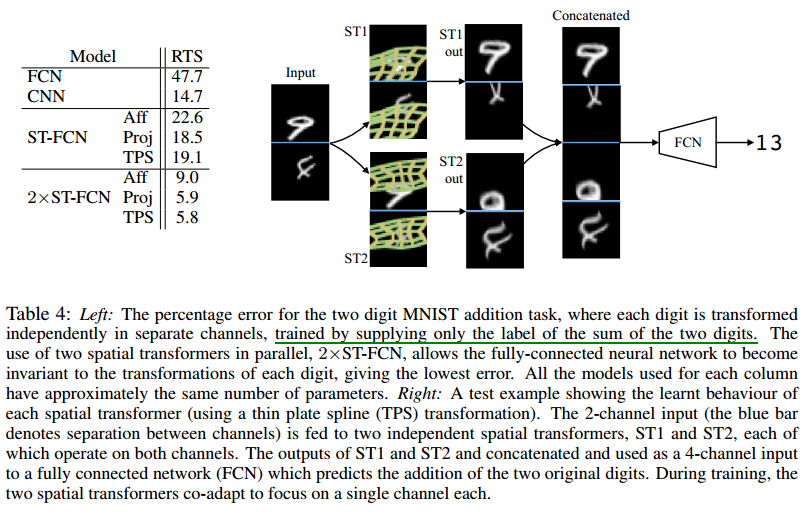• 由于数据比较复杂，FCN的效果很差，添加了 Spatial Transformer之后，错误率显著下降
• CNN有池化层存在所以效果比FCN好
• 2×ST-CNN效果最好
• 从右边可视化的图中可以看到虽然每个输入channel都输入到了两个Spatial Transformer中，但是每个Spatial Transformer都是对其中一个channel作用强，而且这两个Spatial Transformer是互补的，所以最后连接起来之后 4个通道的feature map中有两个是完整的数字，所以识别较为有效。

## 3.5 Co-localisation

Co-localisation ：给一些图片，假设这些图片包含一些目标（也可能不包含），在不使用目标类别标签和目标位置标签的情况下，定位出常见的目标。

• 最下面一行代表在同类别的样本中挑选一张 $I_n$ 做一个随机裁剪，裁剪出的这一块 $I_n^{rand}$ ,认为是目标位置
• 中间这一行代表将同样的样本 $I_n$输入 Spatial Transformer，输出 $I_n^{T}$
• 上面这行代表另选一个样本 $I_m$ 输入Spatial Transformer，输出 $I_m^{T}$
• 以上三个输出分别经过一个映射函数 $e()$ ，这个函数由 LeNet CNN模型提供，以便于将上述三个feature map映射成向量，映射成向量后可以计算两两之间的距离
• 计算 $L1=||e(I_m^T)-e(I_n^T)|_2$ , $L2=||e(I_n^T)-e(I_n^{rand})||_2$ , 训练过程中就是要保证 L1 < L2，L2是一个随机裁剪与经过Spatial Transformer的输出之间的距离，理应大于L1.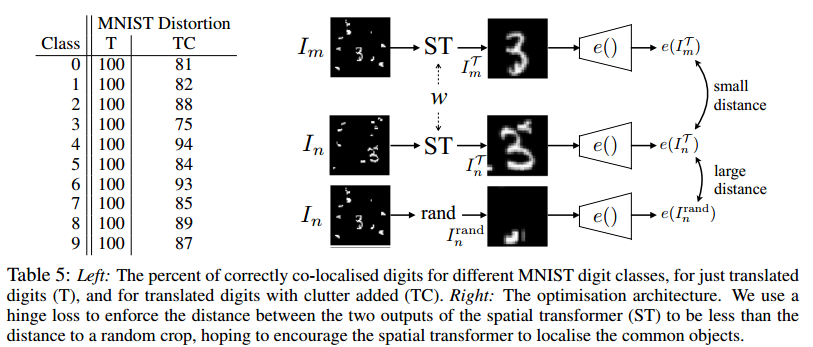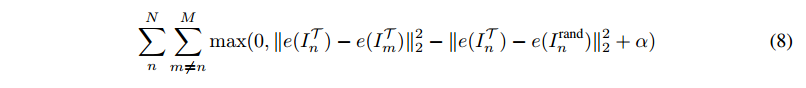$α$ is a margin ,可以称为 裕度，相当于净赚多少。